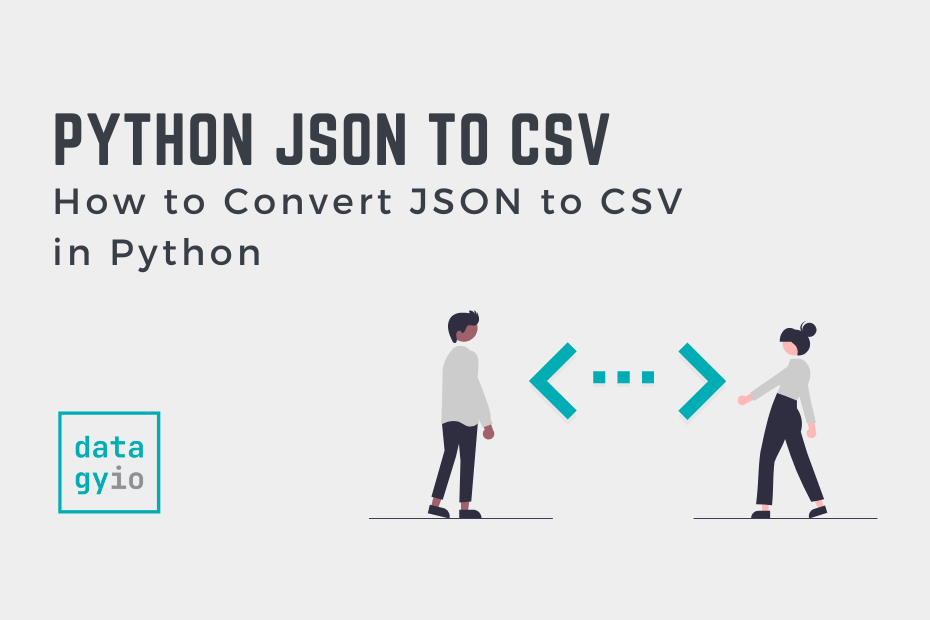# How to Convert JSON to CSV in PythonJavaScript Object Notation (JSON) is one of the most common formats you’ll encounter when working with data – especially web data. Being able to convert the format into other formats, such as CSV, is an important skill. In this tutorial, you’ll learn how to convert data stored in the JSON format into the CSV format using Python.

There are two main ways to accomplish this:

1. Using the popular Pandas library to convert JSON to CSV, and
2. Using only the built-in JSON and CSV libraries

Let’s dive into how to use both of these methods to convert JSON to CSV.

To follow along, we’ll be using a pre-built JSON string. If you’re working with your own string, feel free to use that. However, your results will, of course, vary. Let’s take a look at what the string has:

``````[
{
"Name":"Nik",
"Age":30,
"Active":true
},
{
"Name":"Kate",
"Age":32,
"Active":true
},
{
"Name":"Evan",
"Age":45,
"Active":false
}
]``````

In the pretty-printed string above, we can see that we have three records, each with three fields inside of them. Let’s condense this JSON object and turn it into a string:

``````# Loading a JSON String
json_string = '[{"Name": "Nik", "Age": 30, "Active": true}, {"Name": "Kate", "Age": 32, "Active": true}, {"Name": "Evan", "Age": 45, "Active": false}]'``````

Now that we have our JSON string loaded, let’s see how we can use Pandas to convert it to a CSV file.

## Use Pandas to Convert JSON to CSV

Pandas makes it easy to convert a JSON file to CSV using the `pd.read_json()` function, coupled with the `.to_csv()` method.

Let’s see how we can use Pandas to convert a JSON string to a CSV file:

``````# Using Pandas to Convert a JSON String to a CSV File
import pandas as pd

json_string = '[{"Name": "Nik", "Age": 30, "Active": true}, {"Name": "Kate", "Age": 32, "Active": true}, {"Name": "Evan", "Age": 45, "Active": false}, {"Name": "Kyra", "Age": 43, "Active": true}]'

df.to_csv('file.csv')``````

The following steps convert a JSON string to a CSV file using Python:

1. Import Pandas

Import Pandas using `import pandas as pd`

2. Load the JSON string as a Pandas DataFrame

Load the DataFrame using `pd.read_json(json_string)`

3. Convert the DataFrame to a CSV file

Use the `df.to_csv()` method to convert the DataFrame to a CSV file, by specifying the filename in the method.

## Use Only Python to Convert JSON to CSV

If you’re looking to convert a JSON string into a CSV file without using Pandas, you can use the built-in `json` and `csv` libraries. This allows you to write code that don’t have external dependencies, perhaps making your code more transferable.

Let’s see how we can use just built-in Python library to convert a JSON string to a CSV file:

``````# Convert a JSON String to CSV Using Python
import csv
import json
json_string = '[{"Name": "Nik", "Age": 30, "Active": true}, {"Name": "Kate", "Age": 32, "Active": true}, {"Name": "Evan", "Age": 45, "Active": false}, {"Name": "Kyra", "Age": 43, "Active": true}]'

with open('file.csv', 'w') as f:
writer.writerows(data)``````

The code above has a bit more going on. Let’s break down what the code block is doing:

1. We import both json and csv and load the `json_string`, as before
2. We then load the string into an object, which in this case is a list of dictionaries using the `json.loads()` function
3. We then create a `header` object by accessing the keys of the first item (though, any item would do)
4. We then use a context manager to open a new file
5. We create a new writer `DictWriter` object, passing in the new file and pass in our headers into the `field_names=` parameter
6. We then write the header by using the `.writeheader()` method
7. Finally, we write the rows of the data using the `.writerows()` method

In the final section below, you’ll learn how to convert a JSON file to a CSV file using Python.

## Convert a JSON File to a CSV File Using Pandas

So far, we have explored how to convert a JSON string to a CSV file using Python. However, you may have a JSON file that you want to convert to a CSV file. The simplest way to accomplish this is using Pandas.

The `pd.read_json()` function also accepts a JSON file as its argument, allowing you to load a file directly into a DataFrame. Let’s see how we can replicate our process above using the `pd.read_json()` function:

``````# Convert a JSON File to a CSV File
import pandas as pd

df.to_csv('file.csv')``````

We load the JSON file using the `pd.read_json()` function in the code block above. Then, we convert the DataFrame to a CSV file using the `.to_csv()` method.

## Conclusion

In this tutorial, you learned how to convert a JSON string or file to a CSV file. You first learned the simplest way of doing this, using the Pandas library. Following this, you learned how to accomplish this using only built-in libraries, specifically json and csv. Finally, you learned how to convert a JSON file to a CSV file, using the Pandas method for simplicity.